## April 28, 2015

### Categories in Control

#### Posted by John BaezTo understand ecosystems, ultimately will be to understand networks. - B. C. Patten and M. Witkamp

A while back I decided one way to apply my math skills to help save the planet was to start pushing toward green mathematics: a kind of mathematics that can interact with biology and ecology just as fruitfully as traditional mathematics interacts with physics. As usual with math, the payoffs will come slowly, but they may be large. It’s not a substitute for doing other, more urgent things—but if mathematicians don’t do this, who will?

As a first step in this direction, I decided to study networks.

This May, a small group of mathematicians is meeting in Turin for a workshop on the categorical foundations of network theory, organized by Jacob Biamonte. I’m trying to get us mentally prepared for this. We all have different ideas, yet they should fit together somehow.

Tobias Fritz, Eugene Lerman and David Spivak have all written articles here about their work, though I suspect Eugene will have a lot of completely new things to say, too. Now I want to say a bit about what I’ve been doing with Jason Erbele.

Despite my ultimate aim of studying biological and ecological networks, I decided to start by clarifying the math of networks that appear in chemistry and engineering, since these are simpler, better understood, useful in their own right, and probably a good warmup for the grander goal. I’ve been working with Brendan Fong on electrical ciruits, and with Jason Erbele on control theory. Let me talk about this paper:

• John Baez and Jason Erbele, Categories in control.

Control theory is the branch of engineering that focuses on manipulating open systems—systems with inputs and outputs—to achieve desired goals. In control theory, signal-flow diagrams are used to describe linear ways of manipulating signals, for example smooth real-valued functions of time. Here’s a real-world example; click the picture for more details: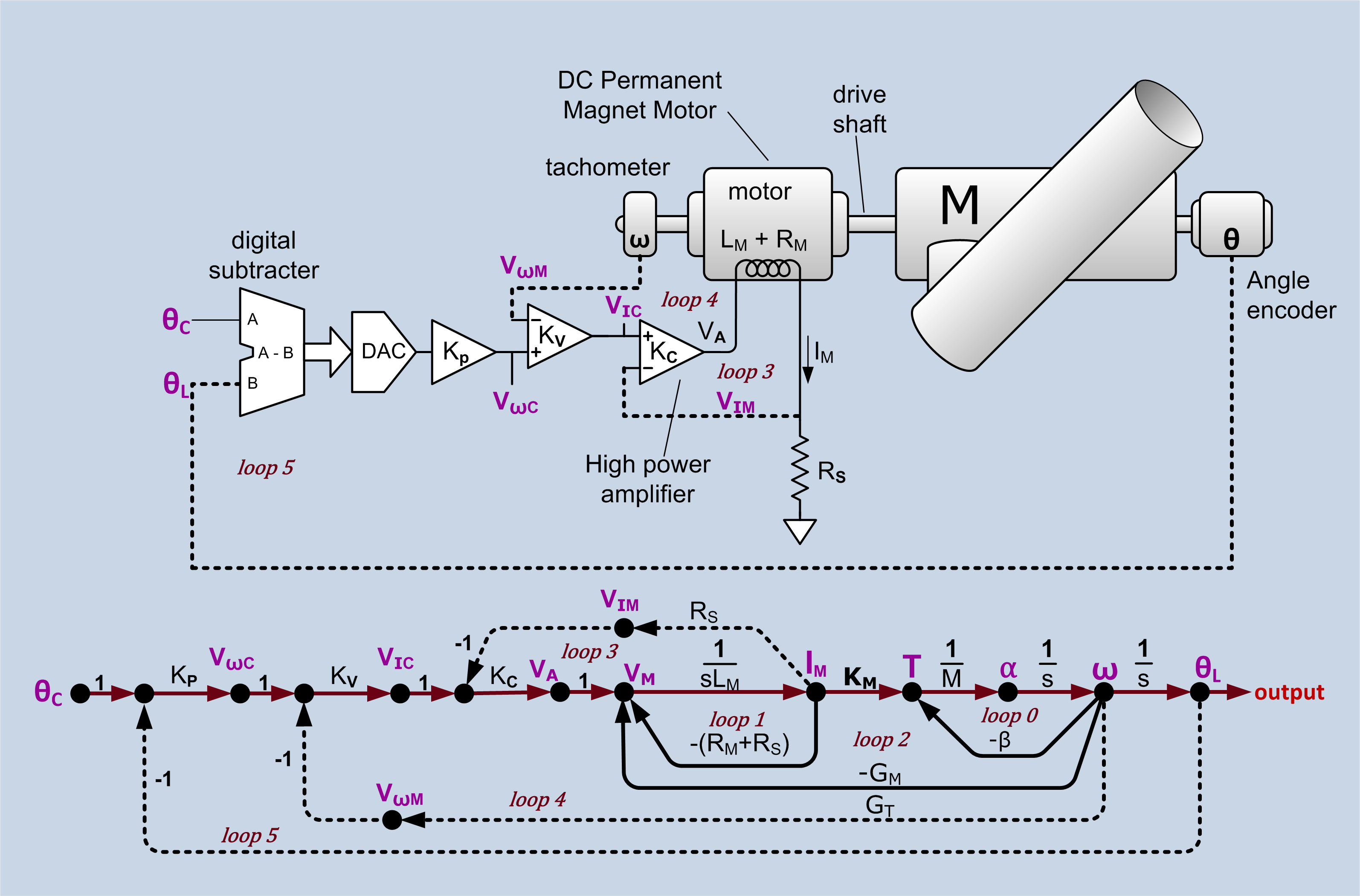For a category theorist, at least, it is natural to treat signal-flow diagrams as string diagrams in a symmetric monoidal category. This forces some small changes of perspective, which I’ll explain, but more important is the question: which symmetric monoidal category?

We argue that the answer is: the category $\mathrm{FinRel}_k$ of finite-dimensional vector spaces over a certain field $k,$ but with linear relations rather than linear maps as morphisms, and direct sum rather than tensor product providing the symmetric monoidal structure. We use the field $k = \mathbb{R}(s)$ consisting of rational functions in one real variable $s.$ This variable has the meaning of differentation. A linear relation from $k^m$ to $k^n$ is thus a system of linear constant-coefficient ordinary differential equations relating $m$ ‘input’ signals and $n$ ‘output’ signals.

Our main goal in this paper is to provide a complete ‘generators and relations’ picture of this symmetric monoidal category, with the generators being familiar components of signal-flow diagrams. It turns out that the answer has an intriguing but mysterious connection to ideas that are familiar in the diagrammatic approach to quantum theory! Quantum theory also involves linear algebra, but it uses linear maps between Hilbert spaces as morphisms, and the tensor product of Hilbert spaces provides the symmetric monoidal structure.

We hope that the category-theoretic viewpoint on signal-flow diagrams will shed new light on control theory. However, in this paper we only lay the groundwork.

### Signal flow diagrams

There are several basic operations that one wants to perform when manipulating signals. The simplest is multiplying a signal by a scalar. A signal can be amplified by a constant factor:

$f \mapsto cf$

where $c \in \mathbb{R}.$ We can write this as a string diagram: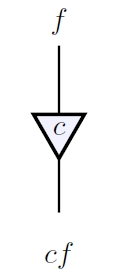Here the labels $f$ and $c f$ on top and bottom are just for explanatory purposes and not really part of the diagram. Control theorists often draw arrows on the wires, but this is unnecessary from the string diagram perspective. Arrows on wires are useful to distinguish objects from their duals, but ultimately we will obtain a compact closed category where each object is its own dual, so the arrows can be dropped. What we really need is for the box denoting scalar multiplication to have a clearly defined input and output. This is why we draw it as a triangle. Control theorists often use a rectangle or circle, using arrows on wires to indicate which carries the input $f$ and which the output $c f.$

A signal can also be integrated with respect to the time variable:

$f \mapsto \int f$

Mathematicians typically take differentiation as fundamental, but engineers sometimes prefer integration, because it is more robust against small perturbations. In the end it will not matter much here. We can again draw integration as a string diagram: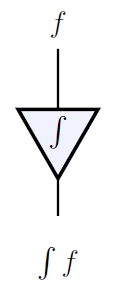Since this looks like the diagram for scalar multiplication, it is natural to extend $\mathbb{R}$ to $\mathbb{R}(s),$ the field of rational functions of a variable $s$ which stands for differentiation. Then differentiation becomes a special case of scalar multiplication, namely multiplication by $s,$ and integration becomes multiplication by $1/s.$ Engineers accomplish the same effect with Laplace transforms, since differentiating a signal $f$ is equivalent to multiplying its Laplace transform

$\displaystyle{ (\mathcal{L}f)(s) = \int_0^\infty f(t) e^{-st} \,dt }$

by the variable $s.$ Another option is to use the Fourier transform: differentiating $f$ is equivalent to multiplying its Fourier transform

$\displaystyle{ (\mathcal{F}f)(\omega) = \int_{-\infty}^\infty f(t) e^{-i\omega t}\, dt }$

by $-i\omega.$ Of course, the function $f$ needs to be sufficiently well-behaved to justify calculations involving its Laplace or Fourier transform. At a more basic level, it also requires some work to treat integration as the two-sided inverse of differentiation. Engineers do this by considering signals that vanish for $t < 0,$ and choosing the antiderivative that vanishes under the same condition. Luckily all these issues can be side-stepped in a formal treatment of signal-flow diagrams: we can simply treat signals as living in an unspecified vector space over the field $\mathbb{R}(s).$ The field $\mathbb{C}(s)$ would work just as well, and control theory relies heavily on complex analysis. In our paper we work over an arbitrary field $k.$

The simplest possible signal processor is a rock, which takes the 'input' given by the force $F$ on the rock and produces as 'output' the rock's position $q.$ Thanks to Newton's second law $F=ma,$ we can describe this using a signal-flow diagram: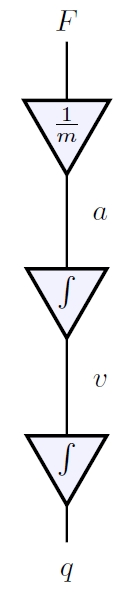Here composition of morphisms is drawn in the usual way, by attaching the output wire of one morphism to the input wire of the next.

To build more interesting machines we need more building blocks, such as addition:

$+ : (f,g) \mapsto f + g$

and duplication:

$\Delta : f \mapsto (f,f)$

When these linear maps are written as matrices, their matrices are transposes of each other. This is reflected in the string diagrams for addition and duplication: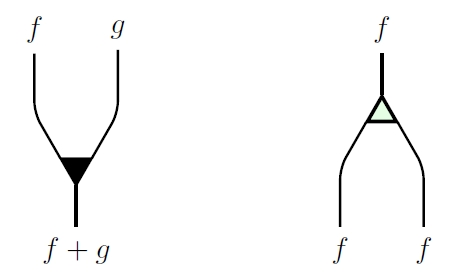The second is essentially an upside-down version of the first. However, we draw addition as a dark triangle and duplication as a light one because we will later want another way to ‘turn addition upside-down’ that does not give duplication. As an added bonus, a light upside-down triangle resembles the Greek letter $\Delta,$ the usual symbol for duplication.

While they are typically not considered worthy of mention in control theory, for completeness we must include two other building blocks. One is the zero map from the zero-dimensional vector space $\{0\}$ to our field $k,$ which we denote as $0$ and draw as follows: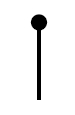The other is the zero map from $k$ to $\{0\},$ sometimes called ‘deletion’, which we denote as $!$ and draw thus: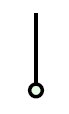Just as the matrices for addition and duplication are transposes of each other, so are the matrices for zero and deletion, though they are rather degenerate, being $1 \times 0$ and $0 \times 1$ matrices, respectively. Addition and zero make $k$ into a commutative monoid, meaning that the following relations hold: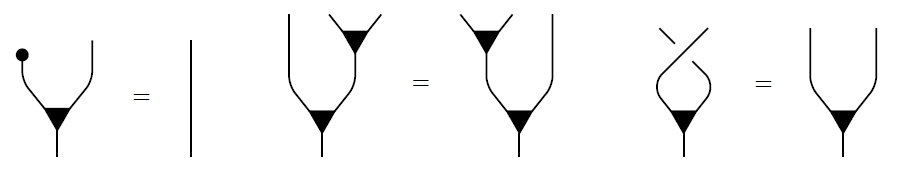The equation at right is the commutative law, and the crossing of strands is the braiding:

$B : (f,g) \mapsto (g,f)$

by which we switch two signals. In fact this braiding is a symmetry, so it does not matter which strand goes over which: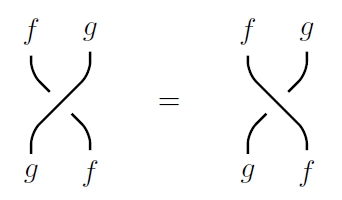Dually, duplication and deletion make $k$ into a cocommutative comonoid. This means that if we reflect the equations obeyed by addition and zero across the horizontal axis and turn dark operations into light ones, we obtain another set of valid equations:There are also relations between the monoid and comonoid operations. For example, adding two signals and then duplicating the result gives the same output as duplicating each signal and then adding the results: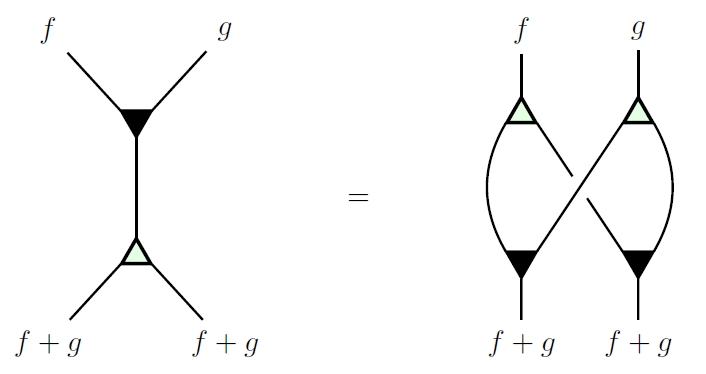This diagram is familiar in the theory of Hopf algebras, or more generally bialgebras. Here it is an example of the fact that the monoid operations on $k$ are comonoid homomorphisms—or equivalently, the comonoid operations are monoid homomorphisms.

We summarize this situation by saying that $k$ is a bimonoid. These are all the bimonoid laws, drawn as diagrams: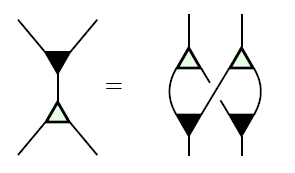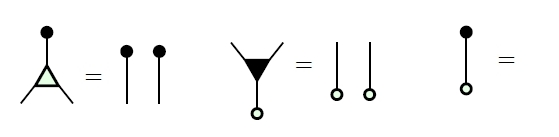The last equation means we can actually make the diagram at left disappear, since it equals the identity morphism on the 0-dimensional vector space, which is drawn as nothing.

So far all our string diagrams denote linear maps. We can treat these as morphisms in the category $\mathrm{FinVect}_k,$ where objects are finite-dimensional vector spaces over a field $k$ and morphisms are linear maps. This category is equivalent to the category where the only objects are vector spaces $k^n$ for $n \ge 0,$ and then morphisms can be seen as $n \times m$ matrices. The space of signals is a vector space $V$ over $k$ which may not be finite-dimensional, but this does not cause a problem: an $n \times m$ matrix with entries in $k$ still defines a linear map from $V^n$ to $V^m$ in a functorial way.

In applications of string diagrams to quantum theory, we make $\mathrm{FinVect}_k$ into a symmetric monoidal category using the tensor product of vector spaces. In control theory, we instead make $\mathrm{FinVect}_k$ into a symmetric monoidal category using the direct sum of vector spaces. In Lemma 1 of our paper we prove that for any field $k,$ $\mathrm{FinVect}_k$ with direct sum is generated as a symmetric monoidal category by the one object $k$ together with these morphisms: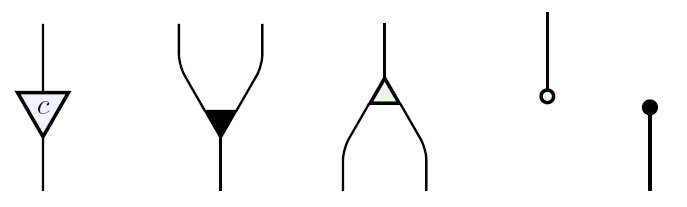where $c \in k$ is arbitrary.

However, these generating morphisms obey some unexpected relations! For example, we have: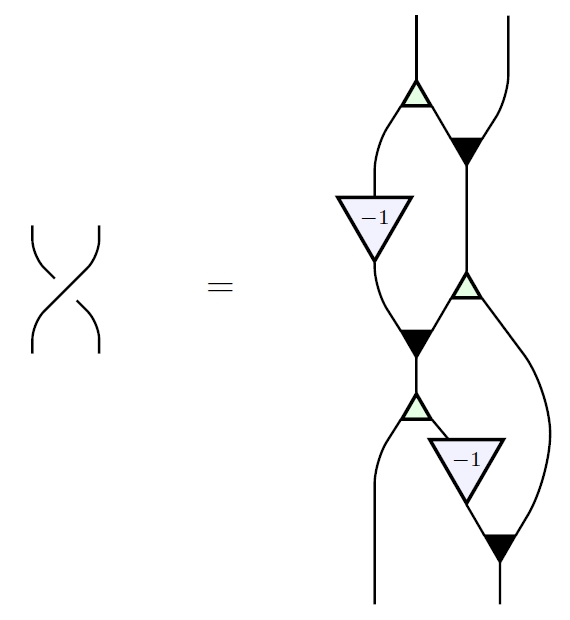Thus, it is important to find a complete set of relations obeyed by these generating morphisms, thus obtaining a presentation of $\mathrm{FinVect}_k$ as a symmetric monoidal category. We do this in Theorem 2. In brief, these relations say:

(1) $(k, +, 0, \Delta, !)$ is a bicommutative bimonoid;

(2) the rig operations of $k$ can be recovered from the generating morphisms;

(3) all the generating morphisms commute with scalar multiplication.

Here item (2) means that $+, \cdot, 0$ and $1$ in the field $k$ can be expressed in terms of signal-flow diagrams as follows: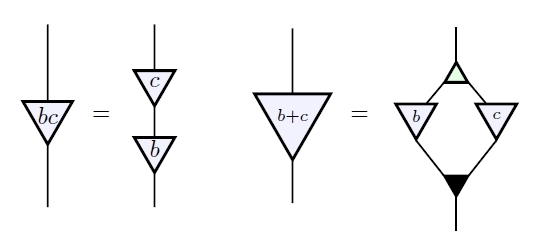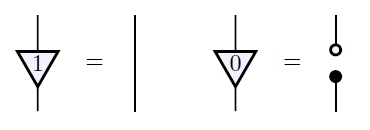Multiplicative inverses cannot be so expressed, so our signal-flow diagrams so far do not know that $k$ is a field. Additive inverses also cannot be expressed in this way. So, we expect that a version of Theorem 2 will hold whenever $k$ is a mere rig: that is, a ‘ring without negatives’, like the natural numbers. The one change is that instead of working with vector spaces, we should work with finitely presented free $k$-modules.

Item (3), the fact that all our generating morphisms commute with scalar multiplication, amounts to these diagrammatic equations: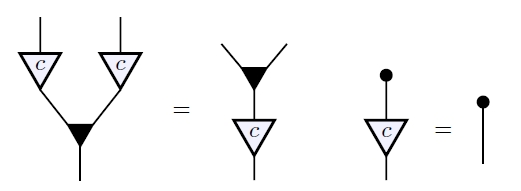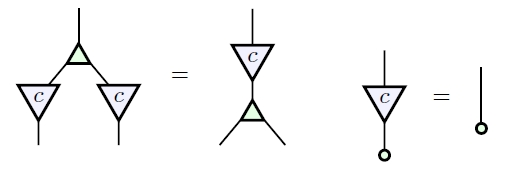While Theorem 2 is a step towards understanding the category-theoretic underpinnings of control theory, it does not treat signal-flow diagrams that include ‘feedback’. Feedback is one of the most fundamental concepts in control theory because a control system without feedback may be highly sensitive to disturbances or unmodeled behavior. Feedback allows these uncontrolled behaviors to be mollified. As a string diagram, a basic feedback system might look schematically like this: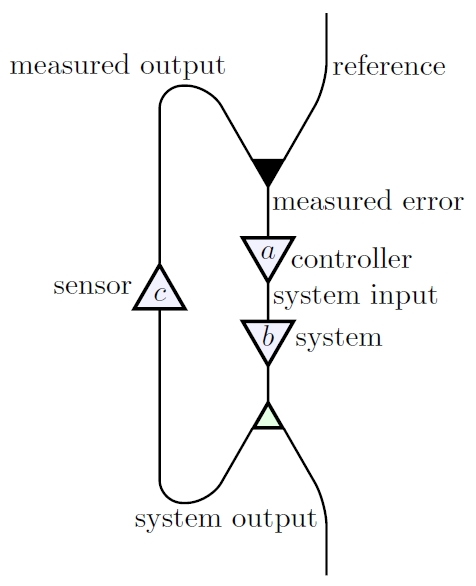The user inputs a ‘reference’ signal, which is fed into a controller, whose output is fed into a system, which control theorists call a ‘plant’, which in turn produces its own output. But then the system’s output is duplicated, and one copy is fed into a sensor, whose output is added (or if we prefer, subtracted) from the reference signal.

In string diagrams—unlike in the usual thinking on control theory—it is essential to be able to read any diagram from top to bottom as a composite of tensor products of generating morphisms. Thus, to incorporate the idea of feedback, we need two more generating morphisms. These are the ‘cup’: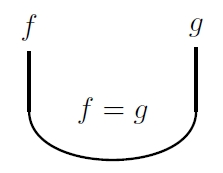and ‘cap’: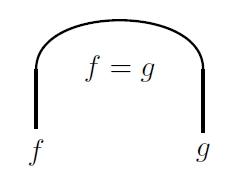These are not maps: they are relations. The cup imposes the relation that its two inputs be equal, while the cap does the same for its two outputs. This is a way of describing how a signal flows around a bend in a wire.

To make this precise, we use a category called $\mathrm{FinRel}_k.$ An object of this category is a finite-dimensional vector space over $k,$ while a morphism from $U$ to $V,$ denoted $L : U \rightharpoonup V,$ is a linear relation, meaning a linear subspace

$L \subseteq U \oplus V$

In particular, when $k = \mathbb{R}(s),$ a linear relation $L : k^m \to k^n$ is just an arbitrary system of constant-coefficient linear ordinary differential equations relating $m$ input variables and $n$ output variables.

Since the direct sum $U \oplus V$ is also the cartesian product of $U$ and $V,$ a linear relation is indeed a relation in the usual sense, but with the property that if $u \in U$ is related to $v \in V$ and $u' \in U$ is related to $v' \in V$ then $c u + c'u'$ is related to $c v + c'v'$ whenever $c,c' \in k.$

We compose linear relations $L : U \rightharpoonup V$ and $L' : V \rightharpoonup W$ as follows:

$L'L = \{(u,w) \colon \; \exists\; v \in V \;\; (u,v) \in L \; and \; (v,w) \in L'\}$

Any linear map $f : U \to V$ gives a linear relation $F : U \rightharpoonup V,$ namely the graph of that map:

$F = \{ (u,f(u)) : u \in U \}$

Composing linear maps thus becomes a special case of composing linear relations, so $\mathrm{FinVect}_k$ becomes a subcategory of $\mathrm{FinRel}_k.$ Furthermore, we can make $\mathrm{FinRel}_k$ into a monoidal category using direct sums, and it becomes symmetric monoidal using the braiding already present in $\mathrm{FinVect}_k.$

In these terms, the cup is the linear relation

$\cup : k^2 \rightharpoonup \{0\}$

given by

$\cup \; = \; \{ (x,x,0) : x \in k \} \; \subseteq \; k^2 \oplus \{0\}$

while the cap is the linear relation

$\cap : \{0\} \rightharpoonup k^2$

given by

$\cap \; = \; \{ (0,x,x) : x \in k \} \; \subseteq \; \{0\} \oplus k^2$

These obey the zigzag relations: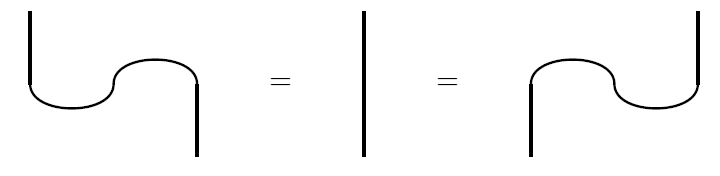Thus, they make $\mathrm{FinRel}_k$ into a compact closed category where $k,$ and thus every object, is its own dual.

Besides feedback, one of the things that make the cap and cup useful is that they allow any morphism $L : U \rightharpoonup V$ to be ‘plugged in backwards’ and thus ‘turned around’. For instance, turning around integration: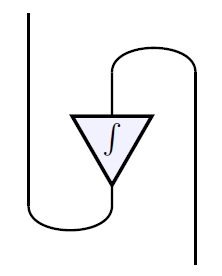we obtain differentiation. In general, using caps and cups we can turn around any linear relation $L : U \rightharpoonup V$ and obtain a linear relation $L^\dagger : V \rightharpoonup U,$ called the adjoint of $L,$ which turns out to given by

$L^\dagger = \{(v,u) : (u,v) \in L \}$

For example, if $c \in k$ is nonzero, the adjoint of scalar multiplication by $c$ is multiplication by $c^{-1}$: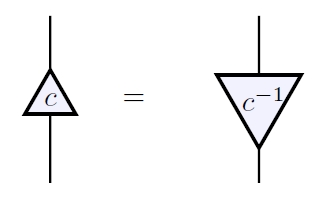Thus, caps and cups allow us to express multiplicative inverses in terms of signal-flow diagrams! One might think that a problem arises when when $c = 0,$ but no: the adjoint of scalar multiplication by $0$ is

$\{(0,x) : x \in k \} \subseteq k \oplus k$

In Lemma 3 we show that $\mathrm{FinRel}_k$ is generated, as a symmetric monoidal category, by these morphisms: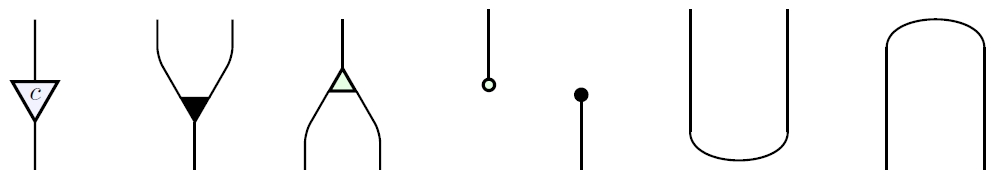where $c \in k$ is arbitrary.

In Theorem 4 we find a complete set of relations obeyed by these generating morphisms,thus giving a presentation of $\mathrm{FinRel}_k$ as a symmetric monoidal category. To describe these relations, it is useful to work with adjoints of the generating morphisms. We have already seen that the adjoint of scalar multiplication by $c$ is scalar multiplication by $c^{-1},$ except when $c = 0.$ Taking adjoints of the other four generating morphisms of $\mathrm{FinVect}_k,$ we obtain four important but perhaps unfamiliar linear relations. We draw these as ‘turned around’ versions of the original generating morphisms:

Coaddition is a linear relation from $k$ to $k^2$ that holds when the two outputs sum to the input:

$+^\dagger : k \rightharpoonup k^2$

$+^\dagger = \{(x,y,z) : \; x = y + z \} \subseteq k \oplus k^2$Cozero is a linear relation from $k$ to $\{0\}$ that holds when the input is zero:

$0^\dagger : k \rightharpoonup \{0\}$

$0^\dagger = \{ (0,0)\} \subseteq k \oplus \{0\}$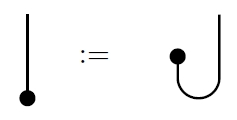Coduplication is a linear relation from $k^2$ to $k$ that holds when the two inputs both equal the output:

$\Delta^\dagger : k^2 \rightharpoonup k$

$\Delta^\dagger = \{(x,y,z) : \; x = y = z \} \subseteq k^2 \oplus k$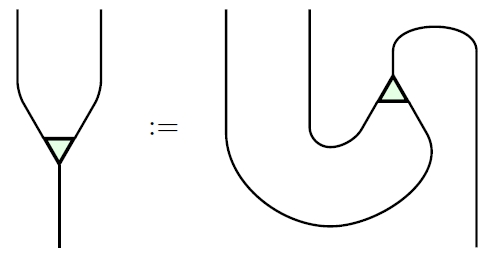Codeletion is a linear relation from $\{0\}$ to $k$ that holds always:

$!^\dagger : \{0\} \rightharpoonup k$

$!^\dagger = \{(0,x) \} \subseteq \{0\} \oplus k$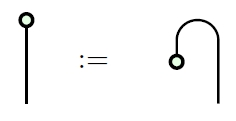Since $+^\dagger,0^\dagger,\Delta^\dagger$ and $!^\dagger$ automatically obey turned-around versions of the relations obeyed by $+,0,\Delta$ and $!,$ we see that $k$ acquires a second bicommutative bimonoid structure when considered as an object in $\mathrm{FinRel}_k.$

Moreover, the four dark operations make $k$ into a Frobenius monoid. This means that $(k,+,0)$ is a monoid, $(k,+^\dagger, 0^\dagger)$ is a comonoid, and the Frobenius relation holds: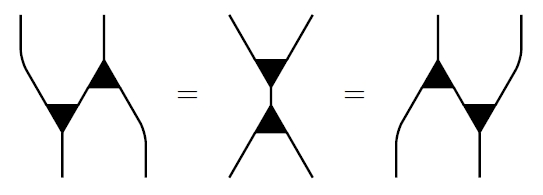All three expressions in this equation are linear relations saying that the sum of the two inputs equal the sum of the two outputs.

The operation sending each linear relation to its adjoint extends to a contravariant functor

$\dagger : \mathrm{FinRel}_k \to \mathrm{FinRel}_k$

which obeys a list of properties that are summarized by saying that $\mathrm{FinRel}_k$ is a †-compact category. Because two of the operations in the Frobenius monoid $(k, +,0,+^\dagger,0^\dagger)$ are adjoints of the other two, it is a †-Frobenius monoid.

This Frobenius monoid is also special, meaning that comultiplication (in this case $+^\dagger$) followed by multiplication (in this case $+$) equals the identity: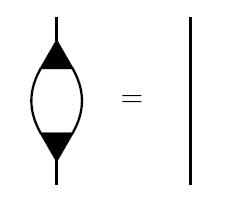This Frobenius monoid is also commutative—and cocommutative, but for Frobenius monoids this follows from commutativity.

Starting around 2008, commutative special †-Frobenius monoids have become important in the categorical foundations of quantum theory, where they can be understood as ‘classical structures’ for quantum systems. The category $\mathrm{FinHilb}$ of finite-dimensional Hilbert spaces and linear maps is a †-compact category, where any linear map $f : H \to K$ has an adjoint $f^\dagger : K \to H$ given by

$\langle f^\dagger \phi, \psi \rangle = \langle \phi, f \psi \rangle$

for all $\psi \in H, \phi \in K .$ A commutative special †-Frobenius monoid in $\mathrm{FinHilb}$ is then the same as a Hilbert space with a chosen orthonormal basis. The reason is that given an orthonormal basis $\psi_i$ for a finite-dimensional Hilbert space $H,$ we can make $H$ into a commutative special †-Frobenius monoid with multiplication $m : H \otimes H \to H$ given by

$m (\psi_i \otimes \psi_j ) = \left\{ \begin{array}{cl} \psi_i & i = j \\ 0 & i \ne j \end{array}\right.$

and unit $i : \mathbb{C} \to H$ given by

$i(1) = \sum_i \psi_i$

The comultiplication $m^\dagger$ duplicates basis states:

$m^\dagger(\psi_i) = \psi_i \otimes \psi_i$

Conversely, any commutative special †-Frobenius monoid in $\mathrm{FinHilb}$ arises this way.

Considerably earlier, around 1995, commutative Frobenius monoids were recognized as important in topological quantum field theory. The reason, ultimately, is that the free symmetric monoidal category on a commutative Frobenius monoid is $2\mathrm{Cob},$ the category with 2-dimensional oriented cobordisms as morphisms. But the free symmetric monoidal category on a commutative special Frobenius monoid was worked out even earlier: it is the category with finite sets as objects, where a morphism $f : X \to Y$ is an isomorphism class of cospans

$X \longrightarrow S \longleftarrow Y$

This category can be made into a †-compact category in an obvious way, and then the 1-element set becomes a commutative special †-Frobenius monoid.

For all these reasons, it is interesting to find a commutative special †-Frobenius monoid lurking at the heart of control theory! However, the Frobenius monoid here has yet another property, which is more unusual. Namely, the unit $0 : \{0\} \rightharpoonup k$ followed by the counit $0^\dagger : k \rightharpoonup \{0\}$ is the identity: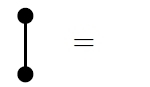We call a special Frobenius monoid that also obeys this extra law extra-special. One can check that the free symmetric monoidal category on a commutative extra-special Frobenius monoid is the category with finite sets as objects, where a morphism $f : X \to Y$ is an equivalence relation on the disjoint union $X \sqcup Y,$ and we compose $f : X \to Y$ and $g : Y \to Z$ by letting $f$ and $g$ generate an equivalence relation on $X \sqcup Y \sqcup Z$ and then restricting this to $X \sqcup Z.$

As if this were not enough, the light operations share many properties with the dark ones. In particular, these operations make $k$ into a commutative extra-special †-Frobenius monoid in a second way. In summary:

$(k, +, 0, \Delta, !)$ is a bicommutative bimonoid;

$(k, \Delta^\dagger, !^\dagger, +^\dagger, 0^\dagger)$ is a bicommutative bimonoid;

$(k, +, 0, +^\dagger, 0^\dagger)$ is a commutative extra-special †-Frobenius monoid;

$(k, \Delta^\dagger, !^\dagger, \Delta, !)$ is a commutative extra-special †-Frobenius monoid.

It should be no surprise that with all these structures built in, signal-flow diagrams are a powerful method of designing processes.

However, it is surprising that most of these structures are present in a seemingly very different context: the so-called ZX calculus, a diagrammatic formalism for working with complementary observables in quantum theory. This arises naturally when one has an $n$-dimensional Hilbert space $H$ with two orthonormal bases $\psi_i, \phi_i$ that are mutually unbiased, meaning that

$|\langle \psi_i, \phi_j \rangle|^2 = \displaystyle{\frac{1}{n}}$

for all $1 \le i, j \le n.$ Each orthonormal basis makes $H$ into commutative special †-Frobenius monoid in $\mathrm{FinHilb}.$ Moreover, the multiplication and unit of either one of these Frobenius monoids fits together with the comultiplication and counit of the other to form a bicommutative bimonoid. So, we have all the structure present in the list above—except that these Frobenius monoids are only extra-special if $H$ is 1-dimensional.

The field $k$ is also a 1-dimensional vector space, but this is a red herring: in $\mathrm{FinRel}_k$ every finite-dimensional vector space naturally acquires all four structures listed above, since addition, zero, duplication and deletion are well-defined and obey all the relations we have discussed. Jason and I focus on $k$ in our paper simply because it generates all the objects $\mathrm{FinRel}_k$ via direct sum.

Finally, in $\mathrm{FinRel}_k$ the cap and cup are related to the light and dark operations as follows: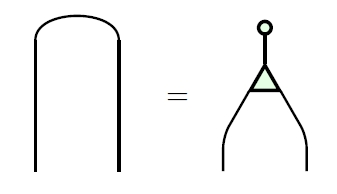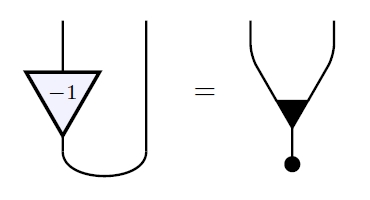Note the curious factor of $-1$ in the second equation, which breaks some of the symmetry we have seen so far. This equation says that two elements $x, y \in k$ sum to zero if and only if $-x = y.$ Using the zigzag relations, the two equations above give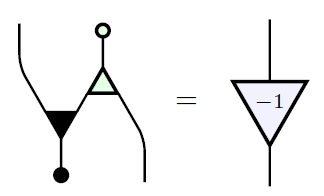We thus see that in $\mathrm{FinRel}_k,$ both additive and multiplicative inverses can be expressed in terms of the generating morphisms used in signal-flow diagrams.

Theorem 4 of our paper gives a presentation of $\mathrm{FinRel}_k$ based on the ideas just discussed. Briefly, it says that $\mathrm{FinRel}_k$ is equivalent to the symmetric monoidal category generated by an object $k$ and these morphisms:

• addition $+: k^2 \rightharpoonup k$ • zero $0 : \{0\} \rightharpoonup k$ • duplication $\Delta: k\rightharpoonup k^2$ • deletion $! : k \rightharpoonup 0$ • scalar multiplication $c: k\rightharpoonup k$ for any $c\in k$ • cup $\cup : k^2 \rightharpoonup \{0\}$ • cap $\cap : \{0\} \rightharpoonup k^2$

obeying these relations:

(1) $(k, +, 0, \Delta, !)$ is a bicommutative bimonoid;

(2) $\cap$ and $\cup$ obey the zigzag equations;

(3) $(k, +, 0, +^\dagger, 0^\dagger)$ is a commutative extra-special †-Frobenius monoid;

(4) $(k, \Delta^\dagger, !^\dagger, \Delta, !)$ is a commutative extra-special †-Frobenius monoid;

(5) the field operations of $k$ can be recovered from the generating morphisms;

(6) the generating morphisms (1)-(4) commute with scalar multiplication.

Note that item (2) makes $\mathrm{FinRel}_k$ into a †-compact category, allowing us to mention the adjoints of generating morphisms in the subsequent relations. Item (5) means that $+, \cdot, 0, 1$ and also additive and multiplicative inverses in the field $k$ can be expressed in terms of signal-flow diagrams in the manner we have explained.

So, we have a good categorical understanding of the linear algebra used in signal flow diagrams!

Now Jason is moving ahead to apply this to some interesting problems… but that’s another story, for later.

Posted at April 28, 2015 10:42 PM UTC

TrackBack URL for this Entry:   https://golem.ph.utexas.edu/cgi-bin/MT-3.0/dxy-tb.fcgi/2820

### Re: Categories in Control

All these diagrams are enough to make my head spin!

It seems to me there’s probably a 2-categorical structure here, where a 2-cell would be an inclusion of one relation inside another. Is this something you’ve looked at?

Unrelatedly, is there a categorical characterization of when a network is “stable”?

Posted by: Tim Campion on April 30, 2015 2:46 PM | Permalink | Reply to this

### Re: Categories in Control

Tim wrote:

All these diagrams are enough to make my head spin!

But it might help to say this. In diagrammatic algebra we love commutative monoids:and we love their upside-down version, cocommutative comonoids:In linear algebra addition gives us a (dark) commutative monoid, and duplication gives us a (light) cocommutative comonoid, so we are very happy.

But when we work with linear relations we can ‘reflect’ any morphism to get one pointing the other way, so we get another (light) commutative monoid and (dark) cocommutative monoid… so we are twice as happy!

There are two main ways a monoid and a comonoid can fit together. They can form a Frobenius monoid:and indeed that’s what happens with the dark operations… and also with the light operations: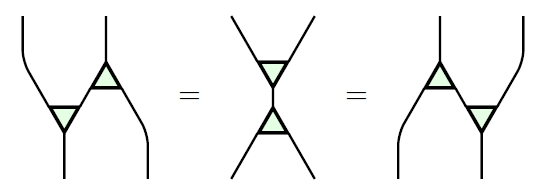Or, they can form a bimonoid: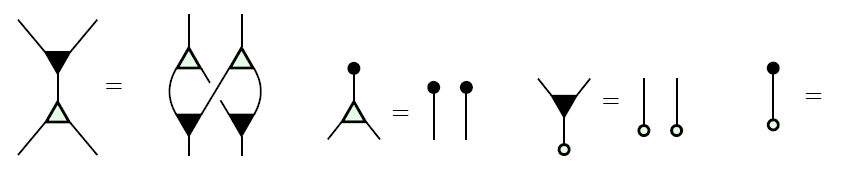and that’s how the dark operations interact with the light ones… but it’s also how the light ones interact with the dark ones: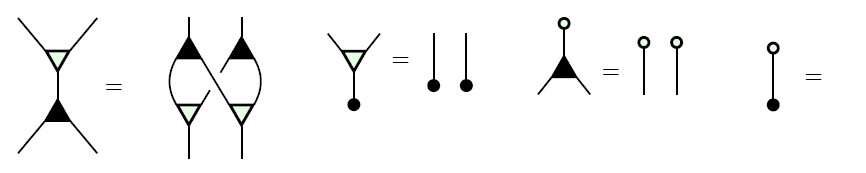So, we’re really in a maximally pleasant context.

There’s a lot to say about why a Frobenius monoids and a bimonoid are the two main ways for a monoid and comonoid to fit together, but I’ll leave that as puzzle.

Posted by: John Baez on April 30, 2015 8:06 PM | Permalink | Reply to this

### Re: Categories in Control

Very interesting , do you know if this aspects are being used in phylogeny ?

Posted by: Helio Jimenez Archundia on October 16, 2019 8:19 PM | Permalink | Reply to this

### Re: Categories in Control

Tim wrote:

It seems to me there’s probably a 2-categorical structure here, where a 2-cell would be an inclusion of one relation inside another. Is this something you’ve looked at?

Indeed the category of linear relations is ‘poset-enriched’, which makes it a 2-category of a specially simple sort.

I haven’t thought much about this. Some people have looked at this idea much more generally: for any regular category you can define a category of relations, which poset-enriched, and indeed forms a pleasant sort of category called an ‘allegory’. But there should be extra beautiful features in the special case we’re considering, or maybe in any abelian category.

Unrelatedly, is there a categorical characterization of when a network is “stable”?

Jason Erbele is studying controllability, observability and stability for signal flow networks. We’ll get back to you on that!

Posted by: John Baez on April 30, 2015 8:49 PM | Permalink | Reply to this

### Re: Categories in Control

One of the nicest papers at the POPL 2015 conference this year was Filippo Bonchi, Pawel Sobocinski, and Fabio Zanasi’s Full Abstraction for Signal Flow Graphs, in which they give an operational semantics for these diagrams (which turns out to be closely related to dataflow programming) and show that it is sound and complete with respect to the categorical semantics.

Posted by: Neel Krishnaswami on May 5, 2015 9:30 AM | Permalink | Reply to this

### Re: Categories in Control

Yes, their work overlaps with ours. Right now I’m updating ‘Categories in control’, adding a section at the end where I explain how their descriptions of $\mathrm{FinVect}_k$ and $\mathrm{FinRel}_k$ are related to ours. I’m also adding a discussion of this new paper:

• Simon Wadsley and Nick Woods, PROPs for linear systems.

which grew out of the old Theorems into Coffee series here on the $n$-Café. Nick is a student of mine, so the sudden revival of this old thread is no coincidence.

Posted by: John Baez on May 5, 2015 7:56 PM | Permalink | Reply to this

### Re: Categories in Control

Neat! You have a typo in the display for composing linear relations.

Can you explain how your example of an “unexpected relation” follows from the basic relations in the presentation?

In string diagrams … it is essential to be able to read any diagram from top to bottom as a composite of tensor products of generating morphisms

Well, the theory of traced monoidal categories uses string diagrams that have “loops” that can’t be broken down into caps and cups. But maybe that wouldn’t capture the same information that you want?

Posted by: Mike Shulman on May 6, 2015 9:25 PM | Permalink | Reply to this

### Re: Categories in Control

Thanks for catching the typo — fixed!

Can you explain how your example of an “unexpected relation” follows from the basic relations in the presentation?

Not offhand; I just know that it must. But you may get your wish. The referee for our paper wants to see some more examples where we use our relations to do stuff. Maybe we could do this one.

Well, the theory of traced monoidal categories uses string diagrams that have “loops” that can’t be broken down into caps and cups. But maybe that wouldn’t capture the same information that you want?

We want a category that contains $FinVect_k$ and is at least traced, and $FinRel_k$ turns out to be a very natural candidate, which is actually compact.

Alternatively we could consider the “free traced monoidal category on the symmetric monoidal category $FinVect_k$”. But I don’t know what that category is like — or even exactly what it means, since I haven’t thought about “freely throwing in traces”.

So, it was easier to work with a category that I knew about already, namely $FinRel_k$, especially since it has a good conceptual interpretation: a morphism in here is “the behavior of a linear machine”. A linear machine is one whose inputs and outputs are related by some set of linear equations. Its behavior, what it “accomplishes”, is that linear relation.

Brendan Fong and I have studied a category that shows up in electrical engineering, where a morphism is an electrical circuit made of linear resistors, inductors and capacitors. This category has a functor to $FinRel_k$ that provides the “semantics” for electrical circuits. In other words, if a circuit is a kind of a machine, we can hit it with this functor and get the behavior of this machine: the relation between its inputs and outputs.

For more, try:

• John Baez and Brendan Fong, A compositional framework for passive linear networks.

Posted by: John Baez on May 7, 2015 4:16 AM | Permalink | Reply to this

### Re: Categories in Control

Alternatively we could consider the “free traced monoidal category on the symmetric monoidal category $FinVect_k$”. But I don’t know what that category is like — or even exactly what it means, since I haven’t thought about “freely throwing in traces”.

I’ve been looking for a reference for freely throwing in traces to a symmetric monoidal category, but all that I’ve found is your comment! In case that anyone knows where this has been done, I’d be glad to hear.

Posted by: Tobias Fritz on June 29, 2015 5:46 PM | Permalink | Reply to this

### Re: Categories in Control

My immediate reaction was “but isn’t $FinVect_k$ already traced, using the good old trace of a matrix?” I think the answer is that it’s traced with respect to the tensor product, but you want to use the direct sum as the monoidal structure. Is that right?

Posted by: Mike Shulman on May 7, 2015 6:16 PM | Permalink | Reply to this

### Re: Categories in Control

Mike wrote:

I think the answer is that it’s traced with respect to the tensor product, but you want to use the direct sum as the monoidal structure. Is that right?

Right, exactly! There’s been a huge amount of work on diagrammatic methods for the symmetric monoidal category $FinVect_k$ with its usual tensor product, since that’s important in quantum mechanics, Feynman diagrams, quantum groups and knot theory, etc.

But we’re exploring a more fundamental and strangely less studied story: diagrammatic methods for the symmetric monoidal category $FinVect_k$ with its direct sum as tensor product. And this turns out to be important in electrical engineering, circuit diagrams, signal flow diagrams and other classical linear systems.

Posted by: John Baez on May 7, 2015 7:36 PM | Permalink | Reply to this

### Re: Categories in Control

Thanks for putting this together. Maybe this will turn into the much needed “Categories for the working Electrical Engineer”. A fresh look at signals and systems will do us working engineers good.

I’m wondering if you have applied this line of thinking yet to digital circuits. I’ve always been baffled by the flip-flop. Take a seemingly simple Boolean circuit, feed the output back to input, and you get a system with memory, and one which has oscillating states. See for example the Wikipedia article on SR NOR latch.

Posted by: Rob MacDonald on May 6, 2015 9:35 PM | Permalink | Reply to this

### Re: Categories in Control

Someday I hope category theory will be of use to (at least a nonempty set of) electrical engineers. So far the flow of information is going the other way: by trying to understand what electrical engineers are doing, we’re getting new ideas about category theory.

I haven’t thought much about digital circuits or even nonlinear analog circuits. A bunch of the abstract framework we’re developing is applicable to those kinds of circuits. But I find it frustrating to develop abstract frameworks without applying them to concrete examples, so I’ve chosen linear analog continuous-time circuits as my primary example to start with. Once I understand that example, I’ll be eager to work on other examples.

So, thanks for giving me something to learn about and think about: how a flip-flop can be used to store information! It should be tractable, since the math is “already understood” to the satisfaction of practitioners. Yet there should still be interesting things to learn, by studying it with the help of category theory and other mathematical power tools.

Posted by: John Baez on May 7, 2015 4:27 AM | Permalink | Reply to this

### Re: Categories in Control

I work on biological control systems and in general these are non-linear. Have I mis-understood or does this only apply to linear control systems?

Posted by: idontgetoutmuch on May 19, 2015 3:38 PM | Permalink | Reply to this

### Re: Categories in Control

This post is about linear systems. Many of the techniques used here also apply to nonlinear systems, but many don’t. My real goal is understanding biology, but it seemed wise to spend some time seeing how traditional linear control theory fits into the framework of modern mathematics before moving on to nonlinear systems.

Posted by: John Baez on May 19, 2015 4:58 PM | Permalink | Reply to this

### Re: Categories in Control

Caveat: I work on inference for the models and not the actual modelling side itself. I found looking at ecological models more accessible and a simple “foxes and rabbits” is non-linear (the interaction term).

You could try e.g. Elements of Mathematical Ecology by Mark Kot.

In my experience, pharmacokinetic / pharmacodynamic models require more background but they may be of interest. Here are some links:

http://www.amstat.org/sections/sbiop/webinars/2012/WebinarSlidesBW11-08-12.pdf

http://www4.stat.ncsu.edu/~davidian/webinar.pdf

I am sure there are plenty of other biological systems that I have neglected.

Posted by: idontgetoutmuch on May 22, 2015 7:07 AM | Permalink | Reply to this

### Re: Categories in Control

idontgetoutmuch wrote:

a simple “foxes and rabbits” is non-linear (the interaction term).

You might like this book, which covers many models of this type:

Mathematically, a ‘Petri net’ is a presentation of a symmetric monoidal category that’s free on some objects and morphisms. Attaching ‘rate constants’ to the morphisms we get a model for the dynanmics of interacting entities. Here’s a Petri net for rabbits and wolves, that gives the model you’re probably talking about: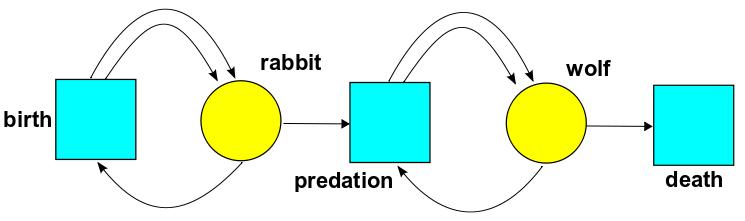Here’s a model of an HIV infection, which I discussed here: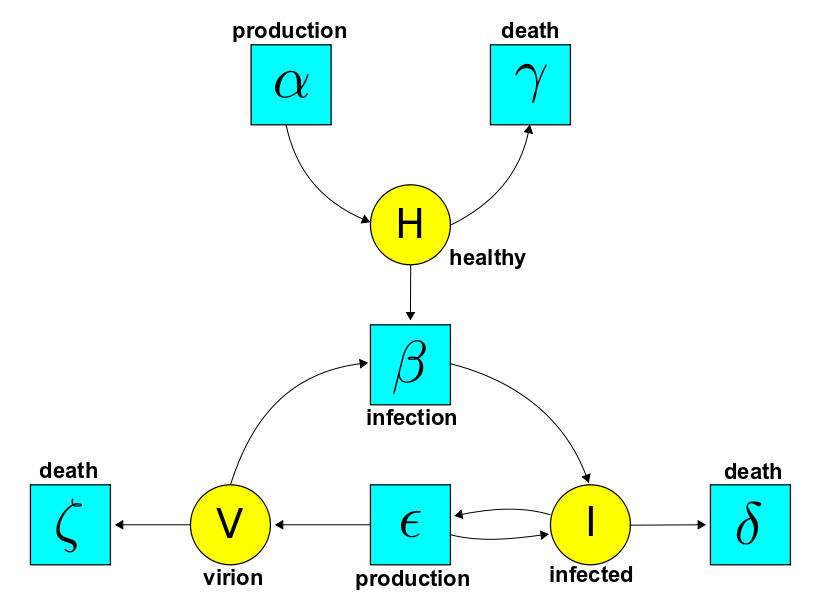So, I’ve been thinking about nonlinear models and category theory from this other viewpoint for a while; connecting those thoughts to the thoughts in this blog article is a project I’d love to tackle soon, especially if I can get a student interested in it!

Posted by: John Baez on May 22, 2015 6:04 PM | Permalink | Reply to this

### Re: Categories in Control

This way you develop applications of category theory looks very interesting and promising, but let me introduce another way, more direct and applicable in real engineering.

First, my definition of simple algebraic gadget, monoid. “Monoid” consists of alphabet A and equivalence eq on the set of all words of this alphabet, such that (stability) if xeqy and x is subword of z then zeqz[x\y], where by z[x\y] I understand replacements of x by y in z (arbitrary number of replacements).

In this way we don’t need to define any operations, everything is encoded in (1) basic free structure, words of alphabet and (2) stable equivalence. In this way we can redefine many other algebraic gadgets, including categories, strict monoidal categories, etc. And such definitions are quite enough to solve equations in such gadgets.

But actually we don’t need that. Instead, using this ideology, we can directly research real engineering systems as algebraic gadgets, because any experienced engineer already knows all practical stable equivalences in his area.

Consider javascript programs, for example (JavaScript is famous programming language). As software engineer I know many stable replacements in such programs. But as algebraist I can forget about semantics of execution of this program and consider the set of such programs, equipped with stable relations, as algebraic gadget. And using stable relations I can solve equations.

If this is interesting for you I could explain some real example, posted here: https://jsfiddle.net/j1p0wso0/1

Most obvious application of this is algebraic verification of engineering systems. It means that the set of requirements to such system can be expressed as equation and system can be certified by proof that this system is solution. In this way we can create non-logical, purely algebraic alternative to logical proof-assistants. The big advantage of this approach is that we don’t need to formalize (set-theoretic or other) semantics of system. All we need is a set of stable equivalences.

But also we can move forward, towards automated solving of such equations, and therefore automated synthesis of systems. “Proof assistance” approach cannot do such the step.

Posted by: Osman on May 20, 2015 10:57 AM | Permalink | Reply to this

### Re: Categories in Control

Sorry, wrong typography.

Again, “Monoid” consists of alphabet $A$ and equivalence $\simeq$ on the set of all words of this alphabet, such that (stability) if $x\, \simeq\, y$ and $x$ is subword of $z$ then $z\, \simeq\, z[x\backslash y]$, where by $z[x\backslash y]$ I understand replacements of $x$ by $y$ in $z$ (arbitrary number of replacements).

Posted by: Osman on May 20, 2015 11:08 AM | Permalink | Reply to this

### Re: Categories in Control

In other words, not only networks (schemes), but any other representations of scientific or engineering information, if these representations are full, can be subjects of algebraic study (through stable equivalences). In fact we can extend category-theoretic notion of doctrine to them and characterize areas of knowledge by these doctrines. I think this is just rebirth of applied math.

Posted by: Osman on June 14, 2015 2:20 PM | Permalink | Reply to this

### Re: Categories in Control

In other words. After 10 or 20 years of thinking “what is proper doctrine for ecosystems?” (electrical, mechanical, control systems… etc.) it can become warm enough in our world to stop theoretizing, because global warming never thinks and never stops.

For reducing this time I suggest another way: somehow ask engineers or scientists what are good equations for describing doctrines/internalizations in their areas. They know, I’m sure, but they don’t understand algebra. But instead of asking “about equations” we can ask “what equivalent replacements you use in your networks in practice?”.

Before that we should describe the notion of equivalence. For example, I did that for computer programs in following way:

Say pieces A and B of code are strongly equivalent iff user that have computer with unlimited memory and unlimited performance can tune these parameters so that he will be unable to observe differences between working programs A and B.

After that I have basis for seekeng for equivalent replacements in computer programs - and for solving equations over programs. And I don’t need to describe semantics and write tons of articles seeking for doctrine.

The same can be done for electricity, mechanics, etc.

Posted by: Osman on June 24, 2015 5:11 PM | Permalink | Reply to this

### Re: Categories in Control

I’d suggest following algorithm of talking to somebody who can help us:

1. Find some engineer/scientist with agile mind and wide experience in certain area (experience of designing something - networks, programs, etc.). For example, programer (if area of choice is software engineering) with 2-3 years of experience is not enough, because cannot make replacements in program with enough accuracy.

2. Sit down together and show how do you solve some equation in string diagrams. Don’t explain categories. Don’t say “composition”, “operation” or “tensor product”. Never say about tensor unit. All you can use is “we can replace this subnetwork by that subnetwork by rule…”.

3. Then ask to show what replacements he does usually in his networks (programs, something else …).

4. Of course our goal is to collect rich enough set of replacements. But it would better if this would become his goal, not only your. So try to demonstrate that you can solve practical equations in that networks, in that area. Show that his intuition for replacements (semantics!) is not needed anymore - everything can be done using just knowledge of certain rules of replacements.

5. In case of success he will quickly show wide and rich set of replacements (as I can do in case of programming) that you will never find from any books or articles.

Posted by: Osman on July 3, 2015 11:39 PM | Permalink | Reply to this

### Re: Categories in Control

I agree that this is a good strategy. I seem to be doing okay looking at diagrams in textbooks, translating them into category theory, and discovering new things to say. But working with cooperative experts would clearly have a lot of advantages.

Posted by: John Baez on July 4, 2015 6:57 AM | Permalink | Reply to this

### Re: Categories in Control

Of course you are okay, because it’s just you. But I’m looking for how to make this work more regular and widely available.

Posted by: Osman on July 4, 2015 8:20 AM | Permalink | Reply to this

### Re: Categories in Control

Posted by: Osman on July 4, 2015 9:18 AM | Permalink | Reply to this

### Re: Categories in Control

I hope something like this happens! I’ve discovered, rather sadly, that I’m not very good at organizing projects like this.

Posted by: John Baez on July 4, 2015 8:51 AM | Permalink | Reply to this

### Re: Categories in Control

John Baez wrote:

I seem to be doing okay looking at diagrams in textbooks, translating them into category theory

My idea is in fact inverse to your. You move domain information into categorical framework, but I suggest to move CT approaches into languages or notations specific for domain. It’s quite possible using ideology of equivalent replacements, including functors, natural transformations and all machinery. I demonstrated the formal idea in my redefinition of monoid above, https://golem.ph.utexas.edu/category/2015/04/categoriesincontrol.html#c049036

So, there are some different features:

1. Go inverse direction.
2. Research not only networks but any other notations where equivalent replacements make sense.
3. Create specific software to make calculations simple.
4. Pursue certain goal: first certify (verify) models of systems and then synthesize them automatically (when software will solve some equations automatically).

I’m sure it’s necessary to make your ideas used widely.

Posted by: Osman on July 7, 2015 12:57 PM | Permalink | Reply to this

### Re: Categories in Control

This question is only tangentially on topic, but what the hell…

I am a working electrical engineer who reads math as a hobby.

I picked up and worked through J.L. Bell’s (excellent!) book “a primer on infinitesimal analysis”.

I was wondering if this theory was extended, and I am using that term loosely, to capture (explain?) Dirac Delta functions (or maybe Distributions would be a better term?)

I found researching the nLab and some papers on Anders Kock’s website that Lawvere/Kock/others have developed a theory of distributions, which I think is related to schwartz distributions. But to be honest, as a category theory-wise dumb EE, who doesn’t know what an inf-groupoid is I am snowed by them!

If anyone reading this blog comes knows of any treatment of Delta functions that is “elementary”, or readable like J.L. Bells’ book, I would love to read it.

This subject fascinates me. It is sort of amazing the calculations that are done with distributions and convolutions by engineers with only a paucity of theory to back them up. for example, pick up say “Continuous and Discrete Signals and Systems” by Soliman and you will find calculations where an infinite train of equally spaced delta functions is convolved with a real signal to produce a sampled output. And these calculations are done (or expected to be) by undergrads who just finished calc 101.

I was so impressed by the Kock/Lawvere presentation of calculus wherein basic theorems are “algebraized” and I have always wondered if there was something similar for delta function (calculations).

Any recommendations welcome.

Cheers

Posted by: Rob MacDonald on August 28, 2015 5:39 PM | Permalink | Reply to this

### Re: Categories in Control

I imagine that people working on nonstandard analysis (that is, infinitesimals) have some fun ways to think about the Dirac delta and other distributions. Maybe Lawvere and Kock have some nice ways too. But, I don’t know any of these approaches. I just learned the usual nonrigorous approach to distributions in my physics classes, together with the usual rigorous approach in my real analysis classes.

Posted by: John Baez on August 31, 2015 5:18 AM | Permalink | Reply to this

### Re: Categories in Control

There are two types of infinitesimals. The infinitesimals used in non-standard analysis à la Robinson are part of a field, which is to say a system of arithmetic in which one can take reciprocals of non-zero elements. These may be called “invertible infinitesimals”.

The other kind of infinitesimals as used in the Kock-Lawvere axioms are very different; particularly, the elements $d$ of the object $D$ that they use satisfy $d^2 = 0$. These are called “nilpotent infinitesmals” (‘nil’ = zero; ‘potent’ = power, i.e. an element which raised to some power is zero). They are definitely not invertible, since if $d^{-1}$ exists then so does $d^{-2}$, which would be an illegal inverse of $0$.

The best kinds of models of synthetic differential geometry or SDG, as developed by Kock, Lawvere, and many others, have both sorts of infinitesimals inside them. The linear differential calculus that involves derivatives, differential forms, integration of linear forms, Stokes’ theorem, and so on – this is developed in terms of nilpotent infinitesimals. My understanding is that Dirac distribution and such is developed in terms of the other kind, invertible infinitesimals. I’m not an expert, but my intuition for the difference is that the Dirac distribution is approximated in finite terms by a function supported over $(-\epsilon/2, \epsilon/2)$ whose average value is $1/\epsilon$, and expressed in infinitesimal terms by a function supported over an interval of infinitesimal length $e$ but with an average value of $1/e$, hence invertible.

Posted by: Todd Trimble on August 31, 2015 12:51 PM | Permalink | Reply to this

### Re: Categories in Control

I’m not an expert either, but my impression is that the invertible infinitesimals in some models of SDG are not as good for this sort of thing as the infinitesimals in NSA. They don’t have as good of a transfer principle, for instance.

Posted by: Mike Shulman on August 31, 2015 6:08 PM | Permalink | Reply to this

### Re: Categories in Control

John wrote:

We use the field $k = \mathbb{R}(s)$ consisting of rational functions in one real variable $s$. A linear relation from $k^m$ to $k^n$ is thus a system of linear constant-coefficient differential equations relating $m$ ‘input’ signals and $n$ ‘output’ signals.

I don’t understand this. Take $m = n = 1$. Then you’re saying that a linear subspace of $k \oplus k = k^2$ is a system of linear constant-coefficient differential equations relating a single input signal and a single output signal.

If I correctly understand what you mean by ‘signal’, this means that a linear subspace of $k^2$ is a system of linear constant-coefficient differential equations in a function $\mathbb{R} \to \mathbb{R}$. Is that right so far?

Whether it’s right or not, maybe you can explain the following example. Let $L$ be the linear subspace of $k \oplus k$ spanned by $(\lambda, \mu)$, where $\lambda, \mu \in \mathbb{R}(s)$ are defined by

$\lambda = \frac{s^2 + 6s - 1}{s + 1}, \qquad \mu = \frac{s + 3}{s^5 + 2}.$

What system of differential equations does $L$ correspond to?

Posted by: Tom Leinster on February 4, 2016 4:46 PM | Permalink | Reply to this

### Re: Categories in Control

Hi, Tom! I’m glad you asked a question, because I think this stuff here is cool.

If I correctly understand what you mean by ‘signal’…

To be precise, I might mean an infinitely differentiable function $f: \mathbb{R} \to \mathbb{R}$ with $f(t) = 0$ for $t \le 0$. That should work fine.

There are other spaces of functions or distributions that would also work. All that really matters is that our set $S$ of signals is an $\mathbb{R}(s)$-module. In the examples that really matter, like the example I just gave, $s$ acts as differentiation:

$sf := f'$

and we can get any rational function of $s$ to act on $S$, via Laplace transforms.

Say we have $\psi \in \mathbb{R}(s)$ and we want it to act on a signal $f \in S$. Then we take the Laplace transform of $f$, multiply it by $\psi$, and take the inverse Laplace transform of the result.

When $\psi(s) = s$, this differentiates $f$.

When $\psi(s) = s^{-1}$, this integrates $f$. More precisely, it gives us the antiderivative $F$ defined by

$F(t) = \int_0^t f(u) \, d u$

If you know Laplace transforms, what I’m saying should sound familiar.

this means that a linear subspace of $k^2$ is a system of linear constant-coefficient differential equations in a function $\mathbb{R} \to \mathbb{R}$. Is that right so far?

Not exactly.

If we take $k = \mathbb{R}(s)$, a subspace of $k \oplus k$ is a linear relation from $k$ to $k$. And this is supposed to describe some collection of linear constant-coefficient differential equations relating one input signal, say $f$, to one output signal, say $g$. If the relation is actually a function, the output $g$ will be a function of the input $f$. That’s the case engineers actually think about. But the other cases turn out to matter for mathematical reasons.

To make life easy on me, you chose the simplest example of a subspace of $k \oplus k$: namely, the one spanned by $(\lambda, \mu)$ where

$\lambda = \frac{s^2 + 6s - 1}{s + 1}, \qquad \mu = \frac{s + 3}{s^5 + 2}.$

Note that this subspace is also spanned by

$(1, \frac{\mu}{\lambda})$

Thus, this linear relation is actually a linear function from $k$ to $k$: the function sending any $f \in k$ to $g = \frac{\mu}{\lambda} f$.

But now, if we have any $k$-module, like our set $S$ of ‘signals’, we can use the same formula to define a function sending an input signal $f \in S$ to an output signal $g \in S$:

$g = \frac{\mu}{\lambda} f$

If your $\lambda$ hadn’t been invertible, this wouldn’t make sense, and I couldn’t express the output as a function of the input. But I could still have written

$\lambda g = \mu f$

and gotten a linear relation between input and output.

What does this relation actually say in your example? Where are the differential equations hiding?

Well, with your $\lambda$ and $\mu$ we have

$\frac{s^2 + 6s - 1}{s + 1} g = \frac{s + 3}{s^5 + 2} f$

or equivalently

$(s^2 + 6s - 1)(s^5 + 2) g = (s+3)(s+1) f$

In the examples that matter, $s$ has the meaning of differentiation, so we get this differential equation relating the output $g$ to the input $f$:

$\left(\frac{d^2}{d t^2} + 6 \frac{d}{d t} - 1\right)\left(\frac{d^2}{d t^2}\right) g = \left(\frac{d}{d t} + 3\right) \left(\frac{d}{d t} + 1\right) f$

Voilà!

Note: I’m using the fact that a linear relation from $k^m$ to $k^n$ gives a linear relation from $S^n$ to $S^m$ whenever $S$ is a $k$-module. I’m just ‘tensoring with $S$’.

I hope that wasn’t too confusing. If the procedure seemed mysterious, please ask another question.

Posted by: John Baez on February 4, 2016 10:28 PM | Permalink | Reply to this

### Re: Categories in Control

One thing that really makes a difference is the space of functions you’re using:

I might mean an infinitely differentiable function $f\colon \mathbb{R} \to \mathbb{R}$ with $f(t) = 0$ for $t \lt 0$.

That restriction is very important! I’d understood that $s$ meant differentiation. But $s$ is also a nonzero scalar in the base field, so the system of differential equations corresponding to the linear subspace $span\{(\lambda, \mu)\}$ of $k \oplus k$ must be the same system that corresponds to $span\{(s\lambda, s\mu)\}$. And that seemed wrong.

For instance, the subspace $span\{(1, 0)\}$ of $k \oplus k$ corresponds to the trivial differential equation $f = 0.$ The subspace $span\{(s, 0)\}$ of $k \oplus k$ corresponds to the not-quite-so-trivial differential equation $\frac{d f}{d t} = 0.$ But since $s$ is a nonzero scalar, $span\{(1, 0)\} = span\{(s, 0)\}$. So in order for your correspondence between subspaces and systems of differential equations to work, we must have $\frac{d f}{d t} = 0 \implies f = 0.$

Of course, that’s usually not the case. But it is if $f$ is restricted to lie in the function space you mention.

Posted by: Tom Leinster on February 5, 2016 1:46 AM | Permalink | Reply to this

### Re: Categories in Control

Yes, electrical engineers have this habit, somewhat mysterious at first, of treating integration as a two-sided inverse to differentiation, as if they didn’t know that functions differing by a constant have the same derivative.

However, their excuse is that they mainly build machines that only start working after you turn them on. Thus, they can assume their signals are zero for $t \le 0.$ This fixes the constant of integration in a way that makes integration a two-sided inverse to differentation.

And the payoff is that their machines, if linear and time-translation-invariant, can be treated as linear relations from $k^m$ to $k^n$, where $k$ is some field of functions in the Laplace transform variable $s$. The easiest case is $k = \mathbb{R}(s)$. But even in this case, they like to think of these rational functions as functions on the complex plane. This lets them prove nice theorems connecting complex analysis to the behavior of their machines.

The only advantage Jason and I have over these electrical engineers is 1) we know category theory and 2) we know that linear functions are a special case of linear relations.

Posted by: John Baez on February 5, 2016 2:14 AM | Permalink | Reply to this

### Re: Categories in Control

Right. And it’s not just that differentiation is being treated as a bijective process. Any differential equation of the form

$a_n f^{(n)} + a_{n-1} f^{(n - 1)} + \cdots + a_1 f' + a_0 f = g$

(where the $a_i$ are constants and $g$ is a “known” function) is assumed to have a unique solution $f$. That’s pretty radical.

We really have to lean on that assumption that our functions $f(t)$ are not only zero for $t \leq 0$, but also infinitely differentiable at $0$. Those engineers must be very sure that their machines start smoothly.

Posted by: Tom Leinster on February 5, 2016 5:12 PM | Permalink | Reply to this

### Re: Categories in Control

Engineers are very realistic and practical about these issues. The small piece of their formalism I’ve described may seem unrealistic taken in isolation, but it’s not used in isolation.

For example, engineers carefully avoid building machines that are described by unstable differential equations — ones where small disturbances amplify over time. Stability can be characterized using the location of the zeros and poles of the rational functions in $s$ that we’ve been discussing. For example,

$f'' + f = 0$

is stable but

$f'' - f = 0$

is not, because the latter has a solution that grows exponentially over time, ultimately because

$s^2 - 1 = 0$

has a root with positive real part.

I suspect that for stable differential equations, your worry become less significant: the solution $f$ of a stable $n$th-order linear ODE should not change dramatically if we change the initial data $f(0), \dots, f^{(n)}(0)$ slightly.

Also, if you’re worried about whether functions really do have infinitely many derivatives, I believe we could also take our space of signals $S$ to consist of tempered distributions supported on the positive half-line. Then $S$ contains very nasty functions like step functions, but any element of $S$ can be differentiated to give another element of $S$.

Anyway, these are just my off-the-cuff guesses, but I know that engineers have thought about these issues a lot more deeply than I have.

Posted by: John Baez on February 6, 2016 3:41 AM | Permalink | Reply to this

### Re: Categories in Control

I’m not sceptical or worried — I’m just getting used to the idea of a world in which all linear differential operators are invertible. It hadn’t occurred to me that such worlds might exist. It’s interesting that they do, especially ones large enough to include tempered distributions.

I admit, I was also a little amused. You memorably explained:

However, their excuse is that they mainly build machines that only start working after you turn them on.

So I imagined a serious, oily piece of machinery, like a two-stroke engine or steam engine or something, being started up…But then I read that it had to start infinitely smoothly, which, with that image in my head, made me smile. But I’m not making any serious or informed objection.

Posted by: Tom Leinster on February 8, 2016 2:17 PM | Permalink | Reply to this

### Re: Categories in Control

Okay! Sorry, these days I react a bit defensively sometimes when mathematicians seem to be criticizing engineers (or, for that matter, vice versa). I’m trying to category theorists — often misunderstood as the ‘the purest of pure’ mathematicians — to talk a bit more to engineers, and vice versa.

In fact, category theorists often seem pretty interested in doing this, since they like to unify things, and they’re always looking for new sources of inspiration.

So far, I’m better at getting ideas from engineering to interest category theorists than vice versa. But I hope that someday the flow of ideas will be two-way…

Posted by: John Baez on February 8, 2016 5:50 PM | Permalink | Reply to this

Post a New Comment